## 7.4.1 Adaptation of Motion Planning Algorithms

All of the components from the general motion planning problem of Formulation 4.1 are included: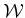,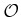,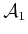,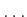,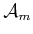,,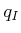, and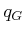. It is assumed that the robot is a collection of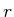links that are possibly attached in loops.

It is assumed in this section that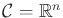. If this is not satisfactory, there are two ways to overcome the assumption. The first is to represent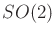and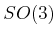as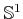and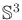, respectively, and include the circle or sphere equation as part of the constraints considered here. This avoids the topology problems. The other option is to abandon the restriction of using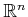and instead use a parameterization ofthat is of the appropriate dimension. To perform calculus on such manifolds, a smooth structure is required, which is introduced in Section 8.3.2. In the presentation here, however, vector calculus onis sufficient, which intentionally avoids these extra technicalities.

Subsections
Steven M LaValle 2020-08-14